The sensitivity of a potentiometer can be increased by

2.  decreasing the length of potentiometer wire

3.  decreasing the current in the potentiometer wire

4.  all of these

Concept Questions :-

Meter bridge and potentiometer
High Yielding Test Series + Question Bank - NEET 2020

Difficulty Level: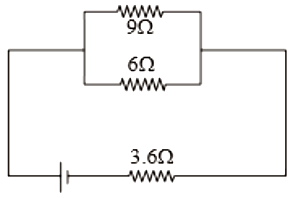1.  36V

2.  18V

3.  9V

4.  Zero

Concept Questions :-

Heating effect of current
High Yielding Test Series + Question Bank - NEET 2020

Difficulty Level:

A current of 1 mA is flowing through a copper wire. How many electrons will pass a given point in one second

1.

2.

3.

4.

Concept Questions :-

Current and current density
High Yielding Test Series + Question Bank - NEET 2020

Difficulty Level:

The drift velocity of free electrons in a conductor is 'v' when a current 'i' is flowing in it. If both the radius and current are doubled, then drift velocity will be :

1.  v

2.

3.

4.  $\frac{\mathrm{v}}{8}$

Concept Questions :-

Current and current density
High Yielding Test Series + Question Bank - NEET 2020

Difficulty Level:

A battery has e.m.f. 4V and internal resistance r. When this battery is connected to an external resistance of 2 ohms, a current of 1 amp. flows in the circuit. How much current will flow if the terminals of the battery are connected directly

1.  1 Amp

2.  2 Amp

3.  4 Amp

4.  Infinite

Concept Questions :-

Emf and terminal voltage
High Yielding Test Series + Question Bank - NEET 2020

Difficulty Level:

Three copper wires have their lengths in the ratio 5:3:1 and their masses are in the ratio 1:3:5. Their electrical resistance will be in the ratio of :

1. 5:3:1

2. 1:3:5

3. 125:15:1

4. 1:15:125

Concept Questions :-

Derivation of Ohm's law
High Yielding Test Series + Question Bank - NEET 2020

Difficulty Level:

The equivalent resistance between A and B is: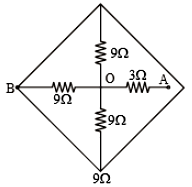1.

2.

3.

4.  $12\mathrm{\Omega }$

Concept Questions :-

Combination of resistors
High Yielding Test Series + Question Bank - NEET 2020

Difficulty Level:

Current I as shown in the circuit will be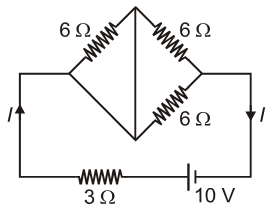1.  10 A

2.

3.

4.

Concept Questions :-

Combination of resistors
High Yielding Test Series + Question Bank - NEET 2020

Difficulty Level:

A meter bridge is set up to determine unknown resistance x using a standard 10 $\Omega$ resistor. The galvanometer shows the null point when the tapping key is at a 52 cm mark. End corrections are 1 cm and 2 cm respectively for end A and B. Then the value of x is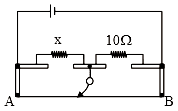1.  10.2$\Omega$

2.  10.6$\Omega$

3.  10.8$\Omega$

3. 11.1$\Omega$

Concept Questions :-

Meter bridge and potentiometer
High Yielding Test Series + Question Bank - NEET 2020

Difficulty Level:

The current through the 5$\mathrm{\Omega }$ resistor is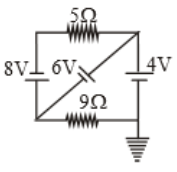(1) 3.2A

(2) 2.8A

(3) 0.8A

(4) 0.2A

Concept Questions :-

Kirchoff's voltage law